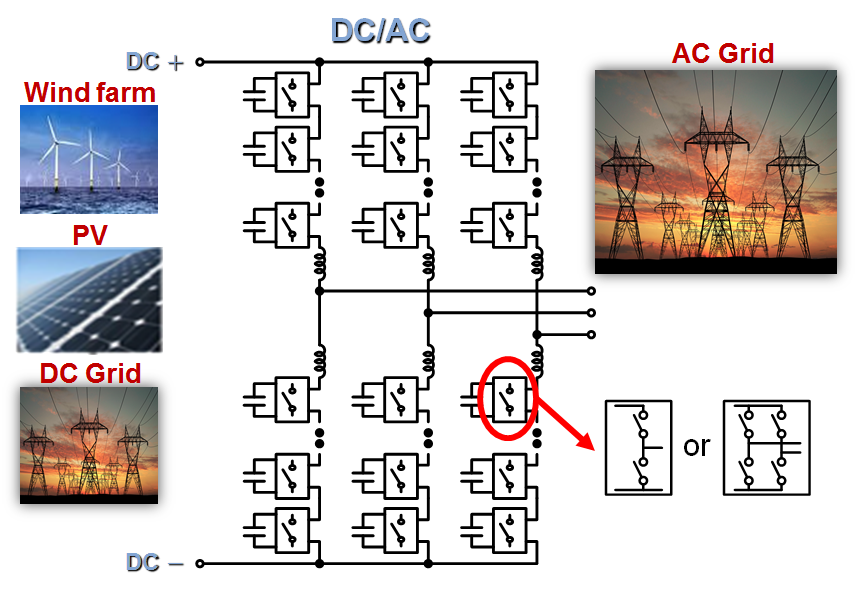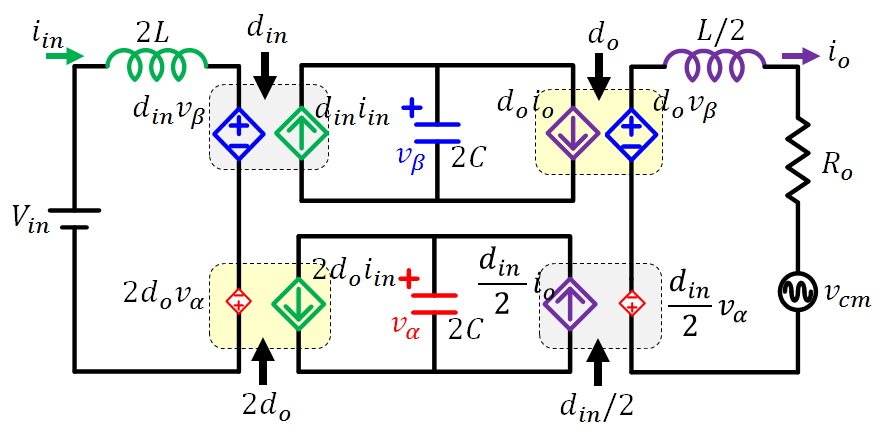LIBRARY

# Decoupled αβ Model of Modular Multilevel Converters (MMCs)

Year: 2017 | Author: Yi-Hsun Hsieh | Paper: D2.11Fig. 1. Schematic of a modular multilevel converter
The modular multilevel converter (MMC), illustrated in Fig. 1 is widely adopted in high-voltage applications because of its simplicity and modularity. However, the current and power flows are very complicated. As a result, methods proposed for capacitor reduction, which is re-quired to store line-frequency related circulating energy, have all gone through complicated mathematical derivation.

Chen Li has proposed a state-plane analysis to visually illustrate the convoluted current and power flows. This analysis was able to explain for the first time the circulating energy related to source and load as well as the circulating energy swapping between capacitors. In addition, he showed that the two different kinds of circulating energy can be decoupled into two orthogonal axes, vα and vβ.

Based on the state-plane analysis, this paper proposes a decoupled αβ model of MMC, as shown in Fig. 2. In this model, the input and output are clearly identified, and vα and vβ represent the total capacitors in one phase. Since vβvα because of the strong DC component, the proposed model clearly shows the major power flow is from Vin through vβ to Ro. Therefore, when input power pin and output power po are not balanced, vβ needs to compensate the difference.

The model also shows that din regulates the average capacitor voltage vβ and controls iin. However, din also generates circulating current on vα. Similarly, do regulates io and generates circulating current on vα at the same time. As a result, when the two controlled current sources in the vα loop are not balanced, the difference becomes the circulating power that swaps between the two capacitors.

The proposed model accurately explains the power flow and the causes for each component of the circulating energy. Therefore, it paves the way for advanced control and a systematic understanding of MMC.Fig. 2. Proposed decoupled αβ model